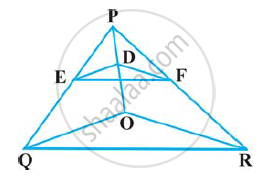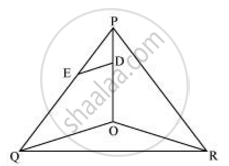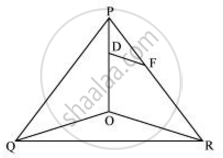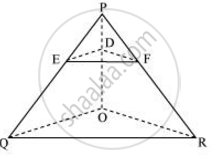Share

# In the following figure, DE || OQ and DF || OR, show that EF || QR - CBSE Class 10 - Mathematics

#### Question

In the following figure, DE || OQ and DF || OR, show that EF || QR#### SolutionIn Δ POQ, DE || OQ

:. (PE)/(EQ) = (PD)/(DO) .... (Basic Proportionality theorem)  (i)In ΔPOR, DF || OR

:. (PF)/(FR) = (PD)/(DO) (Basic Proportionality theorem)  (ii)

From i and ii we obtain

(PE)/(EQ) = (PF)/(FR)

:. EF || QR (Converse of basic proportionally theorem)Is there an error in this question or solution?

#### APPEARS IN

NCERT Solution for Mathematics Textbook for Class 10 (2019 to Current)
Chapter 6: Triangles
Ex. 6.20 | Q: 5 | Page no. 129

#### Video TutorialsVIEW ALL 

Solution In the following figure, DE || OQ and DF || OR, show that EF || QR Concept: Similarity of Triangles.
S